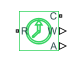# Ideal Rotational Motion Sensor

Motion sensor in mechanical rotational systems

•Libraries:
Simscape / Foundation Library / Mechanical / Mechanical Sensors

## Description

The Ideal Rotational Motion Sensor block represents an ideal mechanical rotational motion sensor, that is, a device that converts an across variable measured between two mechanical rotational nodes into a control signal proportional to acceleration, angular velocity, or position (angle). You can specify the initial angular position (offset) as a block parameter.

The sensor is ideal since it does not account for inertia, friction, delays, energy consumption, and so on.

Connections R and C are mechanical rotational conserving ports that connect the block to the nodes where motion is being monitored. The block positive direction is from port R to port C. This means that the velocity is measured as ω = ωRωC, where ωR, ωC are the absolute angular velocities at ports R and C, respectively. The Measurement reference parameter lets you disable port C and measure with respect to ground.

Connections α, W, and A are physical signal output ports for angular acceleration, velocity, and displacement, respectively. The visibility of these ports is controlled by block parameters. If you enable port α, the block performs additional computations, to measure acceleration based on velocity. To avoid unnecessary model complexity, it is recommended that in each instance of the block you enable only the ports that you actually use for measurement.

The Wrap angle to [0, 2*pi] check box lets you control the angular displacement output range. When selected, it keeps angular displacement within the range from 0 to 2π radians (360 degrees), regardless of the number of revolutions performed by the object and the direction of rotation. When this check box is cleared, the output range is unrestricted.

The figure demonstrates the difference between the two options.In this example, the object is moving at 6 rad/s in the positive direction for the first 5 seconds, and then switches to the negative direction at the same speed. The default angular displacement output (line 1) shows that the object turned forward by 30 rad and then turned back in the negative direction, continuing until –20 rad. If you select the Wrap angle to [0, 2*pi] check box, the output (line 2) stays in the range from 0 to 2π rad.

Selecting the Wrap angle to [0, 2*pi] check box simplifies development of models with complex relationship between model parameters and rotation angle, such as pumps and motors.

## Ports

### Output

expand all

Physical signal output port for angular acceleration.

#### Dependencies

To enable this port, select the Acceleration check box.

Physical signal output port for angular velocity.

#### Dependencies

To enable this port, select the Velocity check box.

Physical signal output port for angular displacement.

#### Dependencies

To enable this port, select the Position check box.

### Conserving

expand all

Mechanical rotational conserving port associated with the sensor positive probe.

Mechanical rotational conserving port associated with the sensor negative (reference) probe.

#### Dependencies

To enable this port, set the Measurement reference parameter to `Difference`.

## Parameters

expand all

Select whether to perform absolute or relative measurements:

• `Difference` — Measure the acceleration, velocity, and position of port R relative to port C.

• `Absolute` — Disable port C and measure the acceleration, velocity, and position of port R with respect to ground (internal reference node).

Selecting this check box exposes output port α, which lets you measure angular acceleration. Exposing port α also involves additional computations, to measure acceleration based on velocity, therefore this port is off by default.

Selecting this check box exposes output port W, which lets you measure angular velocity.

Selecting this check box exposes output port A, which lets you measure position (angular displacement).

When set to `On`, keeps angular displacement output by the sensor within the range from 0 to 2π radians (360 degrees), regardless of the number of revolutions performed by the object and the direction of rotation. When set to `Off`, the output range is unrestricted.

Selecting this check box simplifies development of models with complex relationship between model parameters and rotation angle, such as pumps and motors.

#### Dependencies

To enable this parameter, select the Position check box.

Sensor initial angular position (offset).

#### Dependencies

To enable this parameter, select the Position check box.

## Version History

Introduced in R2007a

expand all